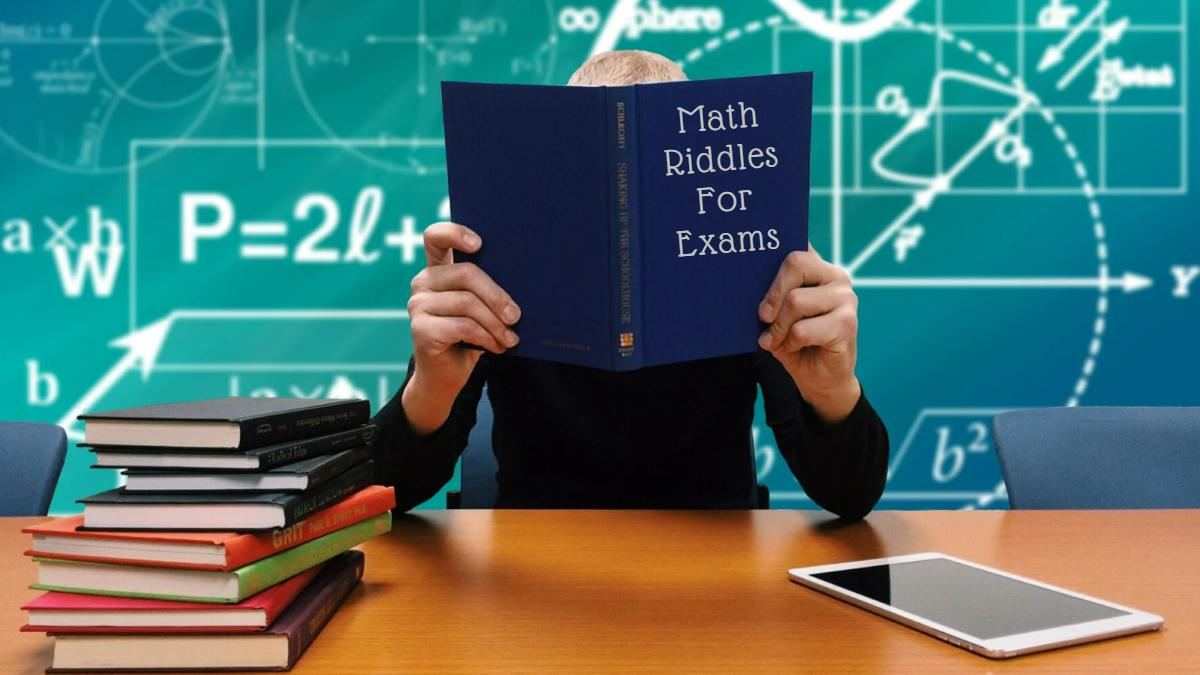These math riddles on algebra will test your knowledge of algebra and help you prepare for any math exam. Test yourself with these math riddles now.There are several reasons why solving math riddles is beneficial for you.

First, they allow you to practice problem-solution strategies. Second, they give you a chance to review basic mathematical concepts. Third, they encourage you to develop critical thinking skills. Finally, they can improve your overall math skills.

This is why we have another set of math riddles from around the web waiting for you.

Are you ready to solve them? We sincerely hope that you are.

So, let’s start!

These 5 Math Riddles Are So Fun To Solve That Even Your Kids Will Love Them!

Math Riddle #1

(x-1)2 = [4√(x-4)]2

Math Riddle #2

A man drives from London to Soho at 8:00 am. His car travels at a constant speed of x km/h. He discovers that he is 50 kilometers from Soho at around 2:00 pm. What is the distance between London and Soho?

Math Riddle #3

Simplify: 4m+5+2m-1

Math Riddle #4

Solve: |- 2 x + 2| - 3 = -3

Math Riddle #5

The sum of two consecutive numbers is 41. What are the numbers?

All the best!

Math Riddle #1
There are two possible values of x.

x = 13 and x = 5.

Math Riddle #2

The distance between London and Soho is (6x + 50) km.

Math Riddle #3

6m + 4

Math Riddle #4

X = 1

Math Riddle #5

The first number is 20 and the second number is 21.

We hope that you liked this math riddle.

Math Riddles: 5 Tough But Fun Math Questions With Answers

Get the latest General Knowledge and Current Affairs from all over India and world for all competitive exams.
खेलें हर किस्म के रोमांच से भरपूर गेम्स सिर्फ़ जागरण प्ले पर